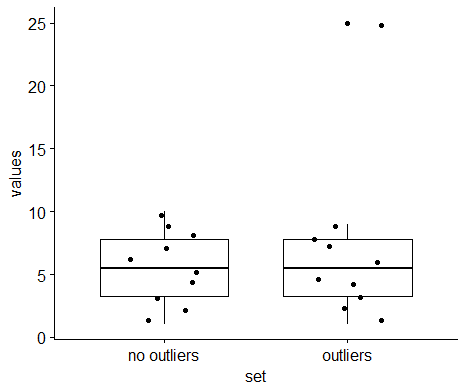# STHDA forum

## • ggpubr » [Solved] Remove outlier points in boxplots with jitterSigned up since: 10/02/2013

Messages: 32

On 08/11/2017 at 08h48
(From a user)

Hi,

When plotting boxplots with outlier points, these outliers are plotted twice when the `add="jitter"` option is set:

Code R :
```
someData <-
data.frame(values=c(1:10, c(1:9, 25)),
set=c(rep("no outliers",10), rep("outliers", 10)))
ggboxplot(data=someData,
x="set",
y="values",
```

The outlying point (with the value 25) is present twice in the picture:Signed up since: 10/02/2013

Messages: 32

On 08/11/2017 at 08h50
Hi,

The ggboxplot() function accepts further arguments to be passed to the `geom_boxplot()` function.

For example, if you want to remove outliers, type this:

Code R :
```
ggboxplot(data=someData,
x="set",
y="values",